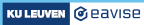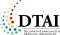3.2. FO in FO(·): Expressions

### 3.2 FO in FO(·)

In IDP-Z3, we express knowledge in FO(·). This language is an extension of classical first-order logic (FO).

We start by discussing expressions, which are the basic building blocks of formulas.

#### 3.2.1 Expressions

Formulas are built out of expressions. Each expression represents a value of a certain type. Expressions are constructed by applying functions or built-in operators to the other expressions of the correct type. For instance, the expression `5 + 1 - 2` represents the value 4 of the built-in type `int`. Because it uses only built-in types and operators, this expression is rigid: its value does not depend on the context in which we consider the expression.

Once our expressions also include symbols that we define ourselves in the vocabulary, they will typically no longer be rigid. For instance, in the vocabulary `AgeVoc`, we previously wrote down the expression `sonsAge() * 2 +1`. This expression is non-rigid: to know which value of type `int` it represents, we need a structure that tells us what the function `sonsAge` looks like. However, the vocabulary does already tell us that expression is correctly typed, since sonsAge() is of type `Int`.

### Exercise

Consider the following vocabulary

For each of the following expressions, indicate whether the expression is correct and, if so, what its type is:

Expression Correct? Type
`foo(g(), h())`
`bar(h())`
`h(bar())`
`bar(foo(bar(h()), i()))`

### Exercise

Consider the following structure for the above vocabulary ExVoc:

What is the value of the expression `bar(foo(bar(h()), i()))` in this structure?

The type of an expression is always completely determined by the vocabulary in which the expression is written. The possible values of this type, on the other hand, may depend on the particular structure in which the expression is considered. For instance, the structure AgeStrucFuncBase we saw earlier defines that the possible values for type Person are Me and MySon, but other structures may assign a different set of possible values to this type, thereby changing the set of possible values that an expression of type Person could have.

So far, we have always declared types in a vocabulary and enumerated their possible values in a structure. It is also possible to enumerate the values of a type immediately in the vocabulary itself: this has the effect of making the type rigid, in the sense that all structures for this vocabulary must now have the same interpretation for this type.

For example, the following are two structures (`DotWS1` and `DotWS2`) for the same vocabulary, each of which assigns a different set of values to the type DayOfTheWeek. This example also illustrates the special notation that is used inside a structure to enumerate predicates (i.e., functions with boolean range): instead of writing each DayOfTheWeek and mapping it to true/false, the enumeration of the predicate weekend simply lists all of the days for which `weekend(day) = true`; all days that are not mentioned are mapped to the value false.

By constrast, the following vocabulary fixes a rigid set of values for the type DayOfTheWeek.

Making the values of the type rigid in this way also allows them to be used in a theory directly, as from the theory's perspective, these values have been declared. For instance, this allows us to move the enumeration of the predicate weekend from a structure to a theory (the "~" symbol represents the boolean operator "not").

###### Made with love (and Hugo) by Joost Vennekens and Simon Vandevelde. :-)